Courses

# Kinetic Theory Of Gases NAT Level - 2

## 10 Questions MCQ Test Topic wise Tests for IIT JAM Physics | Kinetic Theory Of Gases NAT Level - 2

Description
This mock test of Kinetic Theory Of Gases NAT Level - 2 for Physics helps you for every Physics entrance exam. This contains 10 Multiple Choice Questions for Physics Kinetic Theory Of Gases NAT Level - 2 (mcq) to study with solutions a complete question bank. The solved questions answers in this Kinetic Theory Of Gases NAT Level - 2 quiz give you a good mix of easy questions and tough questions. Physics students definitely take this Kinetic Theory Of Gases NAT Level - 2 exercise for a better result in the exam. You can find other Kinetic Theory Of Gases NAT Level - 2 extra questions, long questions & short questions for Physics on EduRev as well by searching above.
*Answer can only contain numeric values
QUESTION: 1

### Air is filled at 60°C in a vessel of open mouth. The vessel is heated to a temperature T so that  1/4th part of the air escapes. Assuming the volume of vessel remaining constant, the value of T is (in °C).

Solution:

M1 = M,
T1 = 60 + 273 = 333 K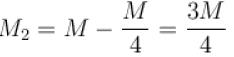(as 1/4 th part of air escapes)
If pressure and volume of gas remain constant then
MT = constant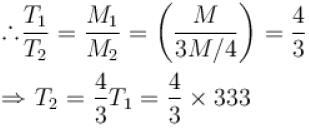⇒ T2 = 444K = 171°C

*Answer can only contain numeric values
QUESTION: 2

### A gas at a certain volume and temperature has pressure 75cm of Hg. If the mass of the gas is doubled at the same volume and temperature, its new pressure in units of cm of Hg level?

Solution: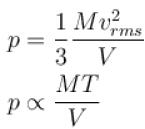At constant volume and temperature, if mass of gas is doubled, then pressure will become twice.

*Answer can only contain numeric values
QUESTION: 3

### A flask contains 10-3 m3 gas. At a temperature, the number of molecule of oxygen are 3 × 1022 . The mass of an oxygen molecule is 5.3 × 10-26 kg and at that temperature, the rms velocity of molecules is 400 m/s. The pressure (in 104 N/m2 ) of the gas in the flask is.

Solution: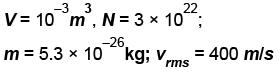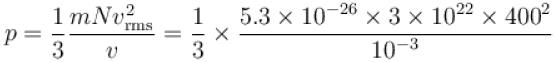p = 8.48 × 104 N/m2

*Answer can only contain numeric values
QUESTION: 4

The root means square speed of hydrogen molecules at 300 K is 1930 m/s. Then the root mean square of oxygen molecules at 900 K will be (in m/s).

Solution: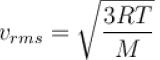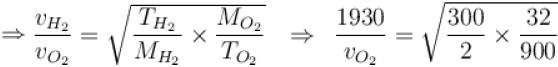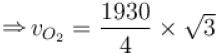= 836 m/s

*Answer can only contain numeric values
QUESTION: 5

At which of the following temperature would the molecules of a gas have twice the average kinetic energy they have at 20°C (in °C)

Solution:

E ∝ T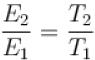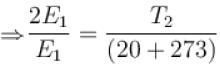⇒ T2 = 293 × 2 = 586 K
⇒ T2 = 313 °C

*Answer can only contain numeric values
QUESTION: 6

The speeds of 5 molecules of a gas (in arbitrary units) are as follows : 2, 3, 4, 5, 6. The root mean square speed for these molecules is.

Solution: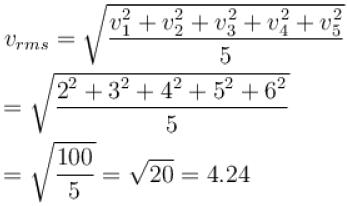*Answer can only contain numeric values
QUESTION: 7

Two identical glass bulbs are inter connected by a thin glass tube. A gas is filled in these bulbs at NTP. If one bulb is placed in ice and another bulb is placed is hot bath, then the pressure of the gas becomes 1.5 times. the temperature of hot bath will be (in °C).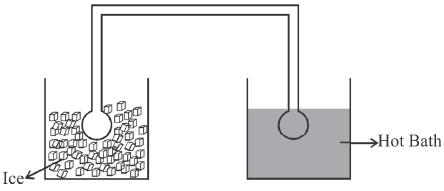Solution:

Quantity of gas in these bulbs is constant i.e.
Initial number of moles is both bulb = Final Number of moles.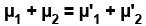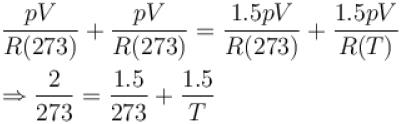⇒ T = 819 K = 546°C

*Answer can only contain numeric values
QUESTION: 8

Hydrogen gas is filled in a balloon at 20°C. If temperature is made 40°C, pressure remaining same, what fraction of hydrogen will come out?

Solution:

As V ∝ T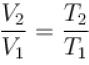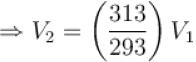Fraction of gas coming out =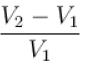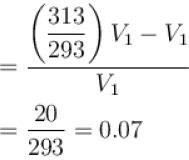*Answer can only contain numeric values
QUESTION: 9

A closed vessel contains  8gm of oxygen and 7gm of nitrogen. The total pressure is 10atm at a given temperature. If now oxygen is absorbed by introducing a suitable absorbent the pressure of the remaining gas (in atm) will be?

Solution:

From Dalton’s law, find pressure of mixture of nitrogen and oxygen
p = p1+ p2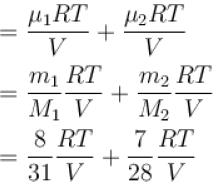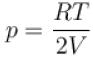...(i)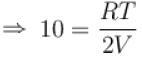when oxygen is absorbed, then for nitrogen let pressure be p
and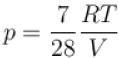...(ii)
From (1) and (2)    p = 5 atm.

*Answer can only contain numeric values
QUESTION: 10

A flask is filled with 13 gm of an ideal gas at 27°C and its temperature is raised to 52°C. The mass of the gas that has to be released to maintains the temperature of the gas in the flask at 52°C and the pressure remaining the same is (in gm).

Solution:

pV ∝ Mass of gas x Temperature
In this problem, pressure and volume remains constant so M1 T1 = M2 T2 = constant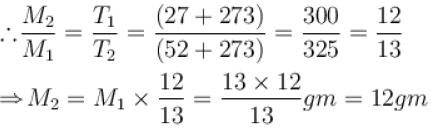i.e., mass of the gas released from the flask = 13 – 12 = 1gm.